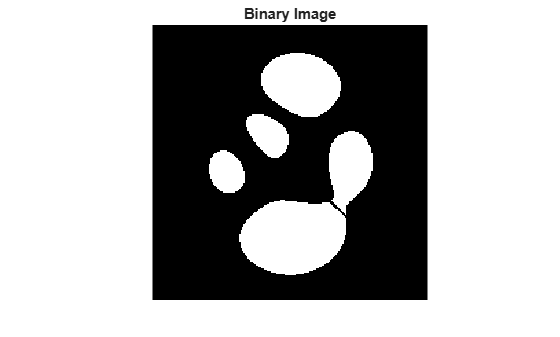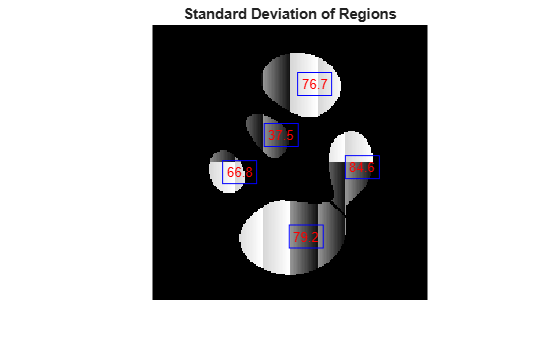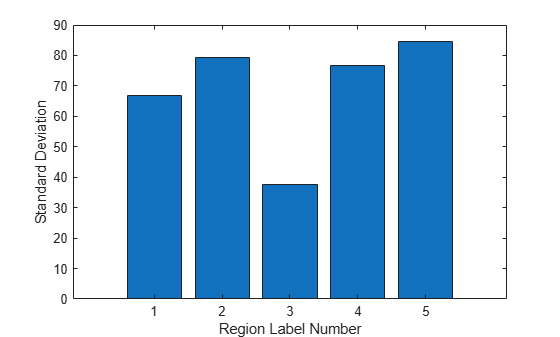# Measuring Regions in Grayscale Images

This example shows how to measure properties of objects in a grayscale image. To accomplish this, first segment the grayscale image to get a binary image of objects. Then, use the `regionprops` function to analyze the original grayscale pixel values corresponding to each object in the binary image.

### Step 1: Create Synthetic Image

Use a helper function, `propsSynthesizeImage`, to create a grayscale image that contains five distinct regions.

```I = propsSynthesizeImage; imshow(I) title('Synthetic Image')```### Step 2: Create a Binary Image

Segment the grayscale image by creating a binary image containing the objects in the original image.

```BW = I > 0; imshow(BW) title('Binary Image')```### Step 3: Calculate Object Properties Using Pixel Values of Grayscale Image

The `regionprops` function supports several properties that can be used with grayscale images, including `'WeightedCentroid'`, `'MeanIntensity'`, `'MinIntensity'`, and `'MaxIntensity'`. These properties use the original pixel values of the objects for their calculations.

For example, you can use `regionprops` to calculate both the centroid and weighted centroid of objects in the image. Note how you pass in the binary image (`BW`) containing your objects and the original grayscale image (`I`) as arguments into `regionprops`.

`s = regionprops(BW,I,{'Centroid','WeightedCentroid'});`

To compare the weighted centroid locations with the unweighted centroid locations, display the original image and then, using the `hold` and `plot` functions, superimpose the centroids on the image.

```imshow(I) title('Weighted (red) and Unweighted (blue) Centroids'); hold on numObj = numel(s); for k = 1 : numObj plot(s(k).WeightedCentroid(1), s(k).WeightedCentroid(2), 'r*') plot(s(k).Centroid(1), s(k).Centroid(2), 'bo') end hold off```### Step 4: Calculate Custom Pixel Value-Based Properties

You can use the `'PixelValues'` property to perform custom calculations based on the pixel values of the original grayscale image. The `'PixelValues'` property returns a vector containing the grayscale values of pixels in a region.

As an example, calculate the standard deviation of each region.

```s = regionprops(BW,I,{'Centroid','PixelValues','BoundingBox'}); imshow(I) title('Standard Deviation of Regions') hold on for k = 1:numObj s(k).StandardDeviation = std(double(s(k).PixelValues)); text(s(k).Centroid(1),s(k).Centroid(2), ... sprintf('%2.1f', s(k).StandardDeviation), ... 'EdgeColor','b','Color','r'); end hold off```This figure shows the standard deviation measurement superimposed on each object in the image. You can also view the results in other ways, for example as a bar plot showing the standard deviation by label number.

```figure bar(1:numObj,[s.StandardDeviation]) xlabel('Region Label Number') ylabel('Standard Deviation')```You can use the plot to determine how to partition the data. For example, the following code identifies objects with a standard deviation lower than 50.

```sStd = [s.StandardDeviation]; lowStd = find(sStd < 50); imshow(I) title('Objects Having Standard Deviation < 50') hold on for k = 1:length(lowStd) rectangle('Position',s(lowStd(k)).BoundingBox,'EdgeColor','y'); end hold off```